# Measuring Line Parameters with an HETG/ACIS-S SpectrumSherpa Threads (CIAO 4.13 Sherpa v1)

## Overview

#### Synopsis:

After having created or downloaded a set of PHA2 and response files (RMFs and ARFs), for a HETG/ACIS-S observation, perform a simple fit to one of the line features present in the spectrum. This thread takes the user through a calculation of the error bars on the line normalizations, positions, and widths, and shows how to calculate the line equivalent widths.

For those wishing to perform their analysis with XSPEC, the PHA2 file must first be split into individual type 1 PHA files, and then grouped to increase the signal-to-noise in each spectral bin. The Grouping a Grating Spectrum CIAO thread shows how to do this, and the procedure is also included within this thread.

You are working with a HETG/ACIS-S data set, and want to begin a simple analysis of the data.

Last Update: 22 Dec 2020 - Updated for CIAO 4.13: adjusting the thread to change when the MEG data was removed and the details of the filtering and grouping; make use of changes to the plot support in CIAO 4.13; add examples of plot_model_component; and simplify the flux calculation by taking advantage of the model parameter added in Sheria 4.12.1.

## Data Preparation

This thread makes use of archival data products for Vela X-1, downloaded from TGcat. They were obtained by performing a "Search by Name" on Vela X-1 (using the SIMBAD name resolver), then choosing "viewfile table" for the target, and clicking the download arrow next to each filename. These files were unzipped and placed in the directory in which the data analysis is to be performed.

Note that we are not considering any background files. For HETG/ACIS-S observations, the background is suppressed by the process of order sorting. That is, an event at a given location along the grating arms must fall into a narrow range of energies (as determined by the CCD) in order for it to be considered a valid dispersed photon. Most background events do not meet these "order sorting" criteria. For this, and many other HETG/ACIS-S observations, the first simple analysis can be performed without reference to the background. (Detailed analysis of low S/N spectra likely would require proper consideration of the background. See the Fitting Grating Data thread for an example of analysis including background data).

Those using either Sherpa or ISIS to perform their analysis can use the data files directly in the analysis; skip to the "Load the Spectrum and Responses" section.

XSPEC users, however, must split and group the spectra before the analysis as described here.

## Load the Spectrum and Responses

Start a Sherpa session:

```unix% sherpa
-----------------------------------------------------
Welcome to Sherpa: CXC's Modeling and Fitting Package
-----------------------------------------------------
Sherpa 4.13.0

Python 3.8.2 (default, Mar 25 2020, 17:03:02)
IPython 7.19.0 -- An enhanced Interactive Python. Type '?' for help.

IPython profile: sherpa
Using matplotlib backend: Qt5Agg

sherpa>
```

We begin by reading the PHA2 file that contains the grating spectra. This file contains 12 spectra, 3 spectral orders each for both positive and negative order HEG and MEG spectra. They are stored in the order: HEG -3, -2, -1, 1, 2, 3; MEG -3, -2, -1, 1, 2, 3. By default, Sherpa will read in all 12 spectral files in this order.

We are only interested, however, in the first-order spectra (positive and negative); therefore, we use a Data Model-type filter to select the third and fourth, ninth, and tenth rows of the PHA2 file (HEG -/+1 and MEG -/+1, respectively).

```sherpa> load_pha("pha2[#row=3,4,9,10]")
statistical errors were found in file 'pha2[#row=3,4,9,10]'
but not used; to use them, re-read with use_errors=True
read background_up into a dataset from file pha2[#row=3,4,9,10]
read background_down into a dataset from file pha2[#row=3,4,9,10]
Multiple data sets have been input: 1-4
```Note

Alternatively, one could use a filter to select all negative and postitive first-order spectra via the TG_M keyword:

```sherpa> load_pha("pha2[TG_M=-1,1]")
```

The load_pha call sets the "file name" of each dataset to the same string, which is not helpful in the plots we will show, so let's set the name field to match the dataset:

```sherpa> get_data(1).name = "pha2[#row=3]"
sherpa> get_data(2).name = "pha2[#row=4]"
sherpa> get_data(3).name = "pha2[#row=9]"
sherpa> get_data(4).name = "pha2[#row=10]"
```

We now read in the associated ARF files and assign them to their corresponding data sets,

```sherpa> load_arf(1, "heg_-1.arf")
```

then do the same for the RMF files.

```sherpa> load_rmf(1, "heg_-1.rmf")
```

## Filtering and Grouping the Data

For the purposes of this analysis, we are interested in fitting the line near 6.4 keV. We restrict the energy range to 5-7 keV for all four spectra using the notice command, since the same energy filter is to be applied to all data set IDs; the notice_id command is available for filtering data sets individually.

```sherpa> notice(5., 7.)
sherpa> show_filter()
Data Set Filter: 1
5.0019-6.9998 Energy (keV)

Data Set Filter: 2
5.0019-6.9998 Energy (keV)

Data Set Filter: 3
5.0044-6.9949 Energy (keV)

Data Set Filter: 4
5.0044-6.9949 Energy (keV)
```

Sherpa allows one to bin the spectra during the analysis session; see the Sherpa thread Changing the grouping scheme of a data set within Sherpa for details.

We bin each of the spectra to have a minimum of 20 counts per energy channel, and to restrict this analysis to the previously-noticed energy range (since the same task is run for all four datasets we use a Python loop):

```sherpa> for i in range(1, 5):
```

The spectra can all be plotted in the same figure using the plot command (chosen to use a logarithmc scale for the Y axis thanks to the set_ylog call). The results are shown in Figure 1:

```sherpa> set_ylog('data')
sherpa> plot("data", 1, "data", 2, "data", 3, "data", 4)
```

### Figure 1: Plot of the +1 and -1 HEG and MEG spectra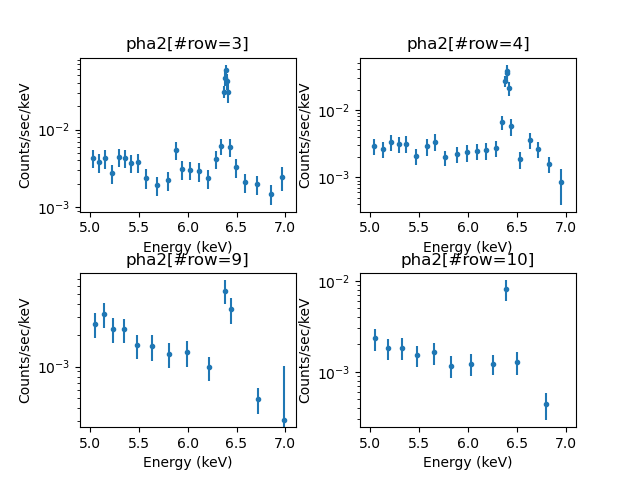### Figure 1: Plot of the +1 and -1 HEG and MEG spectra

The HEG (upper) and MEG (lower) plots with -1 order on the left and and the +1 order on the right. The plots are displayed with a logarithmic scale and have been filtered to the range 5.0-7.0 keV.

Note that the last bin often has a significantly-larger error bar than the preceeding bins, which is because this group does not contain 20 counts. It is up to you to decide whether to keep such bins in your analysis, to filter them out, or to increase the noticed range to add more counts.

Note that associated background spectra (two each for each spectrum, one from either side of the gratings arm) have been read in with the source spectra. We can see this by using list_bkg_ids for one of the datasets:

```sherpa> list_bkg_ids(1)
[1, 2]
```

For this particular dataset the background signal is low (Figure 2):

```sherpa> ungroup(1, bkg_id=1)
sherpa> ungroup(1, bkg_id=2)
sherpa> plot('bkg', 1, 1, 'bkg', 1, 2, yerrorbars=False)
```

### Figure 2: Plot of the background components for the -1 HEG spectrum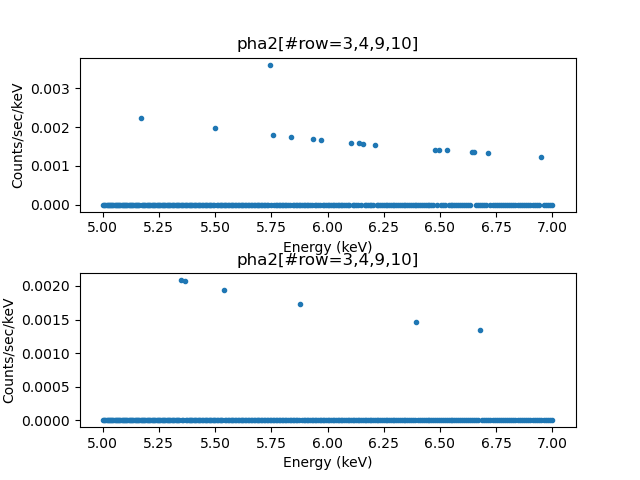### Figure 2: Plot of the background components for the -1 HEG spectrum

Since the background signal is low (there is only one grouped element for each background) we have removed the grouping for the background datasets before plotting the data.

New in CIAO 4.13 is the ability to pass multiple arguments to a plot command in plot, which lets us select both bkg_id components for the first dataset here. Also new is the ability to specify plot options for all the plots, in this case to turn off the display if the Y error bars.

The scaling factor - the number we multiply the background counts by to subtract it from the source region - is calculated by get_bkg_scale:

```sherpa> get_bkg_scale(1, bkg_id=1)
0.12441436912310064
sherpa> get_bkg_scale(1, bkg_id=2)
0.12441436912310064
```

So we can see that in this case the background contribution is small (we should check each dataset individually, but for this source the conclusion holds). Now, unless the subtract command is called, or unless one creates a background model, they will not be part of the fitting process but will cause a warning message to be displayed if not used:

```WARNING: data set 1 has associated backgrounds, but they have not been subtracted, nor have background models been set
```

To avoid this we remove the background components, which requires using a "low-level" set of commands:

```sherpa> for i in range(1, 5):
...:    d = get_data(i)
...:    d.delete_background(2)
...:    d.delete_background(1)
```

The data sets currently being considered in the analysis can be viewed with the show_data, show_bkg, and show_all commands. For example, we can list the datasets to be fitted with the list_data_ids command:

```sherpa> print(list_data_ids())
[1, 2, 3, 4]
```

## Defining the Source Models

We will first fit a broad continuum to the spectra, without any lines. The XSPEC power-law model (xspowerlaw) is chosen for the continuum. It is assigned as the source for data set 1 and given the model name powr; the model name is then used to assign the same source model to the other data sets:

```sherpa> set_source(1, xspowerlaw.powr)
sherpa> set_source(2, powr)
sherpa> set_source(3, powr)
sherpa> set_source(4, powr)
sherpa> show_source()
Model: 1
xspowerlaw.powr
Param        Type          Value          Min          Max      Units
-----        ----          -----          ---          ---      -----
powr.PhoIndex thawed            1           -2            9
powr.norm    thawed            1            0        1e+24

Model: 2
xspowerlaw.powr
Param        Type          Value          Min          Max      Units
-----        ----          -----          ---          ---      -----
powr.PhoIndex thawed            1           -2            9
powr.norm    thawed            1            0        1e+24

Model: 3
xspowerlaw.powr
Param        Type          Value          Min          Max      Units
-----        ----          -----          ---          ---      -----
powr.PhoIndex thawed            1           -2            9
powr.norm    thawed            1            0        1e+24

Model: 4
xspowerlaw.powr
Param        Type          Value          Min          Max      Units
-----        ----          -----          ---          ---      -----
powr.PhoIndex thawed            1           -2            9
powr.norm    thawed            1            0        1e+24
```

## Fitting

The fit statistic is set to be χ2 with the data counts acting as the variance (i.e., a Gaussian approximation to simple Poisson statistics). All data sets are fit simultaneously.

```sherpa> set_stat("chi2datavar")
sherpa> fit()
Datasets              = 1, 2, 3, 4
Method                = levmar
Statistic             = chi2datavar
Initial fit statistic = 8.37325e+08
Final fit statistic   = 393.88 at function evaluation 112
Data points           = 78
Degrees of freedom    = 76
Probability [Q-value] = 2.04873e-44
Reduced statistic     = 5.18263
Change in statistic   = 8.37325e+08
powr.PhoIndex   -1.31318     +/- 0.296211
powr.norm      2.19841e-05  +/- 1.14874e-05
```

At any time, the results of the last fit can be displayed using the show_fit command. The fit yields the following results:

```sherpa> show_fit()
Optimization Method: LevMar
name     = levmar
ftol     = 1.1920928955078125e-07
xtol     = 1.1920928955078125e-07
gtol     = 1.1920928955078125e-07
maxfev   = None
epsfcn   = 1.1920928955078125e-07
factor   = 100.0
numcores = 1
verbose  = 0

Statistic: Chi2DataVar
Chi Squared with data variance.

The variance in each bin is estimated from the data value in that
bin.

If the number of counts in each bin is large, then the shape of
the Poisson distribution from which the counts are sampled tends
asymptotically towards that of a Gaussian distribution, with
variance

sigma(i)^2 = N(i,S) + [A(S)/A(B)]^2 N(i,B)

where N is the number of on-source (and off-source) bins included
in the fit. The background term appears only if an estimate of the
background has been subtracted from the data.

A(B) is the off-source "area", which could be
the size of the region from which the background is extracted, or
the length of a background time segment, or a product of the two,
etc.; and A(S) is the on-source "area". These terms may be defined
for a particular type of data: for example, PHA data sets A(B) to
`BACKSCAL * EXPOSURE` from the background data set and A(S) to
`BACKSCAL * EXPOSURE` from the source data set.

--------
Chi2Gehrels, Chi2ModVar, Chi2XspecVar

Fit:Datasets              = 1, 2, 3, 4
Method                = levmar
Statistic             = chi2datavar
Initial fit statistic = 8.37325e+08
Final fit statistic   = 393.88 at function evaluation 112
Data points           = 78
Degrees of freedom    = 76
Probability [Q-value] = 2.04873e-44
Reduced statistic     = 5.18263
Change in statistic   = 8.37325e+08
powr.PhoIndex   -1.31318     +/- 0.296211
powr.norm      2.19841e-05  +/- 1.14874e-05
```

The results of these fits can then be plotted. Here we choose to display just the data and fits, not the residuals, and overplot on the same plot for easy comparion, resulting in Figure 3:

```sherpa> plot_fit(1, alpha=0.5, ylog=False)
sherpa> plot_fit(2, alpha=0.5, overplot=True)
sherpa> plot_fit(3, alpha=0.5, overplot=True)
sherpa> plot_fit(4, alpha=0.5, overplot=True)
```

### Figure 3: Continuum Fit to the Data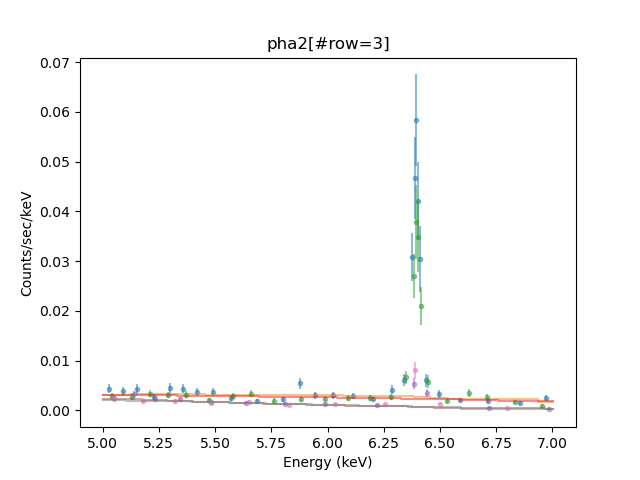### Figure 3: Continuum Fit to the Data

A rather crowded plot, where we can see the difference in the effective area of the HEG and MEG instruments.

New in CIAO 4.13 is the ability to set the transparency of plot objects with the alpha setting.

## Adding Lines to the Model and Fitting Again

We start by creating a Gaussian model component for the feature at 6.4 keV, named g1 and set the Gaussian normalization to 10-4.

```sherpa> one_line = powr + xsgaussian.g1
sherpa> g1.norm = 1e-4
sherpa> for i in range(1, 5):
...:     set_source(i, one_line)
```

which we can fit to all datasets:

```sherpa> fit()
Datasets              = 1, 2, 3, 4
Method                = levmar
Statistic             = chi2datavar
Initial fit statistic = 425.303
Final fit statistic   = 70.9071 at function evaluation 58
Data points           = 78
Degrees of freedom    = 73
Probability [Q-value] = 0.547581
Reduced statistic     = 0.97133
Change in statistic   = 354.396
powr.PhoIndex   -0.679098    +/- 0.324298
powr.norm      6.32886e-05  +/- 3.59468e-05
g1.LineE       6.39408      +/- 0.00123752
g1.Sigma       0.0113278    +/- 0.00174466
g1.norm        0.00018204   +/- 1.02979e-05
```

The improvement in the fit is shown in Figure 4:

```sherpa> plot('fit', 1, 'fit', 2, 'fit', 3, 'fit', 4)
```

### Figure 4: Adding a single line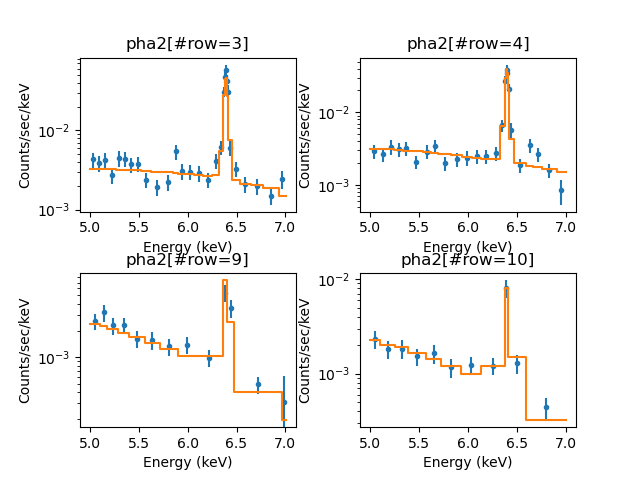### Figure 4: Adding a single line

The -1 orders are in the left column, and +1 orders in the right column. The top row is HEG and bottom row is MEG. The fit is overlaid in orange onto the blue data points.

The MEG spectra do not have very good statistics in the region of interest; therefore, we delete these data sets from the current session before proceeding with the fits.

```sherpa> delete_data(3)
sherpa> delete_data(4)
sherpa> print(list_data_ids())
[1, 2]
```

We refit, to make sure we have the best-fit for just this data:

```sherpa> fit()
Datasets              = 1, 2
Method                = levmar
Statistic             = chi2datavar
Initial fit statistic = 57.338
Final fit statistic   = 56.3026 at function evaluation 25
Data points           = 54
Degrees of freedom    = 49
Probability [Q-value] = 0.220493
Reduced statistic     = 1.14903
Change in statistic   = 1.0354
powr.PhoIndex   -0.664451    +/- 0.384379
powr.norm      6.32152e-05  +/- 4.28647e-05
g1.LineE       6.39384      +/- 0.00122531
g1.Sigma       0.0111641    +/- 0.00170327
g1.norm        0.000189783  +/- 1.15816e-05
```

We can see how this plot (Figure 5) compares to Figure 4:

```sherpa> plot('fit', 1, 'fit', 2)
```

### Figure 5: Restricting to just the HEG data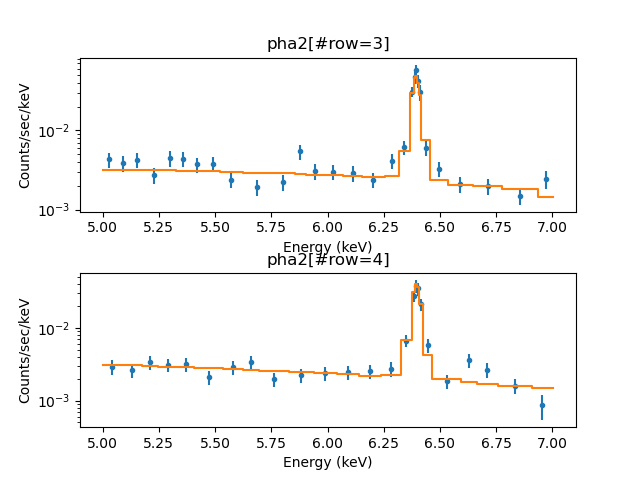### Figure 5: Restricting to just the HEG data

The -1 HEG order is in the upper plot and the +1 HEG order is in the lower plot. The fit is overlaid in orange.

## Examine the Fit Results

We see that we have a good fit to the spectra, including a line component. We can now explore the errors in the line parameters using the conf command to run the Sherpa confidence routines. As is common in X-ray astronomy, we search for the 90% confidence level values for one interesting parameter (i.e., the variation of the parameter of interest that when refitting the remaining unfrozen parameters yields a change in the χ2 value of 2.706). This corresponds to an error bar of 1.64σ (1σ being the 68% confidence interval), and set this as the default error value in the confidence routines via the set_conf_opt command. We then run conf for the three parameters of each of the Gaussian model components: line energy, line width, and line normalization.

```sherpa> set_conf_opt("sigma", 1.64)
sherpa> conf(g1.linee, g1.sigma, g1.norm)
g1.LineE lower bound:   -0.00202748
g1.norm lower bound:    -1.90041e-05
g1.Sigma lower bound:   -0.00307553
g1.LineE upper bound:   0.00201722
g1.norm upper bound:    1.90147e-05
g1.Sigma upper bound:   0.00274967
Datasets              = 1, 2
Confidence Method     = confidence
Iterative Fit Method  = None
Fitting Method        = levmar
Statistic             = chi2datavar
confidence 1.64-sigma (89.8995%) bounds:
Param            Best-Fit  Lower Bound  Upper Bound
-----            --------  -----------  -----------
g1.LineE          6.39384  -0.00202748   0.00201722
g1.Sigma        0.0111641  -0.00307553   0.00274967
g1.norm       0.000189783 -1.90041e-05  1.90147e-05
```

We see that the line position is determined to approximately 0.002 keV, i.e., an equivalent velocity of 80 km/s. This is essentially the limit of the HEG spectral resolution.

The line width is <0.011 keV (i.e., <560 km/sec), but is likely >0.006 keV (i.e., >280 km/sec). Again, these are near the limits of the capabilities of the HEG detector, but far better than the limits one could place with CCD detectors.

Finally, we see that the line normalization is determined to within +/-10%.

We can now plot the data, fits, and residuals (i.e., four separate plots), all on one figure. Since the plot titles overlap each other, and other plot elements, Matplotlib commands are used to clear the title for the bottom two plots (Figure 6).

```sherpa> plot("fit", 1, "fit", 2, "delchi", 1, "delchi", 2)
sherpa> for ax in plt.gcf().axes[2:]:
...:    ax.set_title('')
```

### Figure 6: Fit and Residuals to the Spectral Line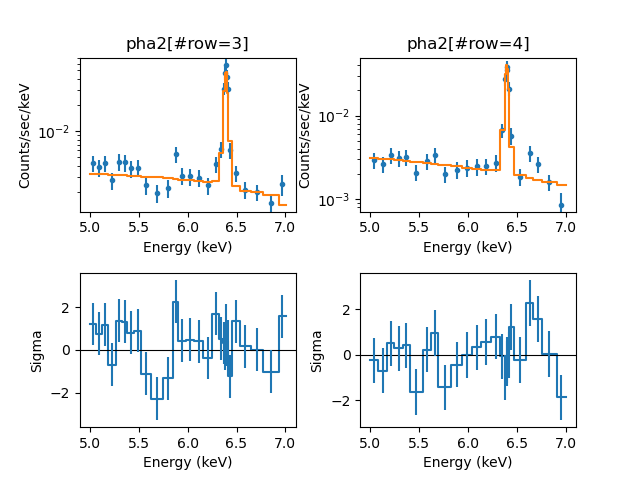### Figure 6: Fit and Residuals to the Spectral Line

The HEG -1 order (left) and +1-order (right).

To explore whether adding another, broader line component changes the results any, we create a second Gaussian component, and call it g2. We then define a model consisting of a power-law plus two Gaussian components, and apply it to both data sets. We change the normalization of the second Gaussian to be somewhat smaller, then fit the data.

```sherpa> full_model = one_line + xsgaussian.g2
sherpa> g2.norm = 1.e-4
sherpa> set_model(1, full_model)
sherpa> set_model(2, full_model)
sherpa> fit()
Datasets              = 1, 2
Method                = levmar
Statistic             = chi2datavar
Initial fit statistic = 155.024
Final fit statistic   = 46.6665 at function evaluation 341
Data points           = 54
Degrees of freedom    = 46
Probability [Q-value] = 0.444864
Reduced statistic     = 1.01449
Change in statistic   = 108.358
powr.PhoIndex   1.87358      +/- 2.07566
powr.norm      0.00435071   +/- 0.0149659
g1.LineE       6.39391      +/- 0.00123494
g1.Sigma       0.00996355   +/- 0.00192428
g1.norm        0.000181852  +/- 1.20256e-05
g2.LineE       6.50072      +/- 0.0745407
g2.Sigma       0.357067     +/- 0.150552
g2.norm        0.000128375  +/- 9.45378e-05

sherpa> conf()
g1.Sigma lower bound:   -0.0035222
g1.norm lower bound:    -1.96287e-05
g1.LineE lower bound:   -0.002048
g1.Sigma upper bound:   0.00297262
g2.LineE lower bound:   -0.131233
powr.norm lower bound:  -0.00421581
g1.LineE upper bound:   0.00203515
g2.Sigma lower bound:   -0.163709
g1.norm upper bound:    1.96349e-05
powr.PhoIndex lower bound:      -2.06799
powr.PhoIndex upper bound:      5.18098
g2.LineE upper bound:   0.168003
g2.norm lower bound:    -8.79802e-05
^[[Ag2.norm upper bound:        0.000227131
g2.Sigma upper bound:   0.31987
powr.norm +: WARNING: The confidence level lies within (1.667857e-01, 1.667808e-01)
powr.norm upper bound:  0.162433
Datasets              = 1, 2
Confidence Method     = confidence
Iterative Fit Method  = None
Fitting Method        = levmar
Statistic             = chi2datavar
confidence 1.64-sigma (89.8995%) bounds:
Param            Best-Fit  Lower Bound  Upper Bound
-----            --------  -----------  -----------
powr.PhoIndex      1.87358     -2.06799      5.18098
powr.norm      0.00435071  -0.00421581     0.162433
g1.LineE          6.39391    -0.002048   0.00203515
g1.Sigma       0.00996355   -0.0035222   0.00297262
g1.norm       0.000181852 -1.96287e-05  1.96349e-05
g2.LineE          6.50072    -0.131233     0.168003
g2.Sigma         0.357067    -0.163709      0.31987
g2.norm       0.000128375 -8.79802e-05  0.000227131
```

The inclusion of the second line component does not greatly affect the conclusions about the parameters of the first line component. The line centroid is the same with comparable error bars. The uncertainty on the line width has increased slightly, as has the uncertainty on the line normalization.

We plot the results for this fit in a manner identical to the one Gaussian line fit (Figure 7).

```sherpa> plot("fit", 1, "fit", 2, "delchi", 1, "delchi", 2)
sherpa> for ax in plt.gcf().axes[2:]:
...:    ax.set_title('')
```

### Figure 7: HEG +/-1 Order Two Gaussian Component Fit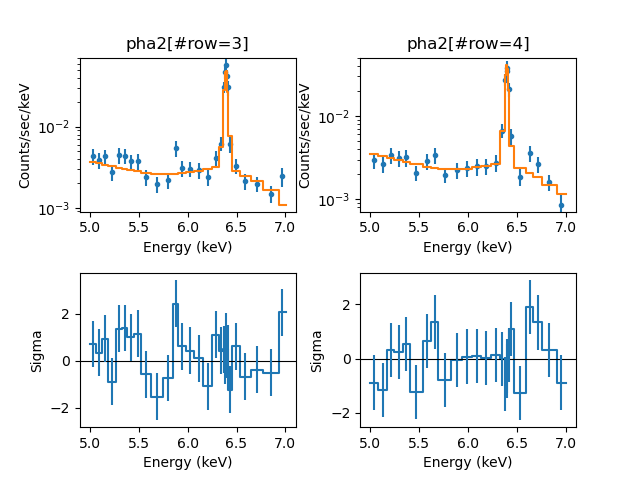### Figure 7: HEG +/-1 Order Two Gaussian Component Fit

The HEG -1-order (left) and +1-order (right).

We can use the plot_model_component function to display the individual components of the fit, lathough for PHA data it requires manually including the instrument response using get_response as shown below:

```sherpa> rsp1 = get_response(1)
sherpa> plot_fit(1)
sherpa> plot_model_component(1, rsp1(g1), overplot=True)
sherpa> plot_model_component(1, rsp1(g2), overplot=True)
sherpa> plot_model_component(1, rsp1(powr), overplot=True)
sherpa> plt.ylim(1e-4, 1e-1)
```

### Figure 8: The line components to the HEG -1 order fit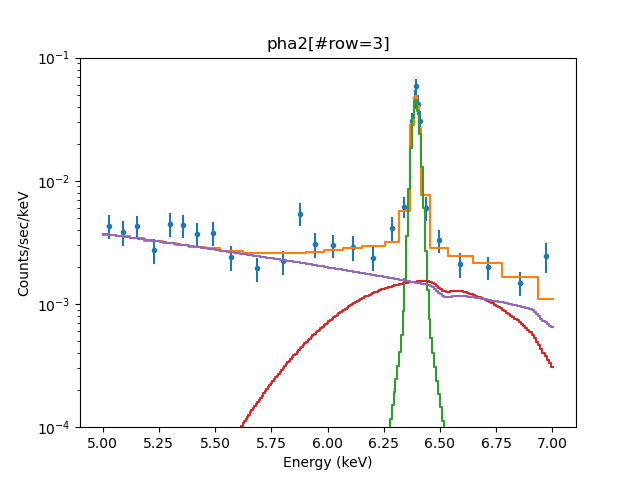### Figure 8: The line components to the HEG -1 order fit

The green (g1) and red (g2) lines show the models for just the line components. We cam see that the g1 line fits the narrow emission line, and the g2 component is much broader. The purple line shows the powr powerlaw component.

Note that plot_model_component displays the ungrouped model (that is, it uses the native energy resolution of the isntrument model) whereas the plot_fit function displays the grouped model data.

Given that the extra component adds little to the description of the data, we drop it from the model and refit the data with a single line, resulting in the same parameters from before.

```sherpa> set_model(1, one_line)
sherpa> set_model(2, one_line)
sherpa> fit()
Datasets              = 1, 2
Method                = levmar
Statistic             = chi2datavar
Initial fit statistic = 115.157
Final fit statistic   = 56.3026 at function evaluation 93
Data points           = 54
Degrees of freedom    = 49
Probability [Q-value] = 0.220493
Reduced statistic     = 1.14903
Change in statistic   = 58.8548
powr.PhoIndex   -0.664451    +/- 0.384379
powr.norm      6.32152e-05  +/- 4.28646e-05
g1.LineE       6.39384      +/- 0.00122531
g1.Sigma       0.0111641    +/- 0.00170327
g1.norm        0.000189783  +/- 1.15816e-05
```

## Calculate the Equivalent Width of a Line and Continuum Flux

Rather than merely presenting the line normalizations, we can instead calculate the line equivalent width. The line equivalent width is an often used measure for the line strength relative to the underlying continuum. Sherpa has a function for evaluating the equivalent width, eqwidth. The required inputs are the source model absent the line feature of interest and the source model with the line feature of interest.

Optionally, the data set number for which the equivalent width will be calculated can also be given as an input, but here as we apply the same model and parameters to both data sets, this is not necessary. There are also optional lo and hi arguments for restricting the calculation of the equivalent width to a subset of the full energy or wavelength range of a data set.

```sherpa> print(1000 * eqwidth(powr, powr + g1))
875.0804657620754
```

The units of the equivalent width are the same as the units of the x-axis, in this case, keV. Thus the line equivalent width is ~875 eV, which indicates a very strong line. That is, there is as nearly as much flux in the line as there is in the continuum over the 6.0-7.0 keV region.

We can verify this by calculating fluxes directly, using the calc_photon_flux and calc_energy_flux commands. We first calculate these fluxes from the full model (continuum plus line) over just the 6.0-7.0 keV range. The returned fluxes are in units of photons/cm2/sec and ergs/cm2/sec, respectively.

```sherpa> pflux_all = calc_photon_flux(6., 7.)
sherpa> eflux_all = calc_energy_flux(6., 7.)
sherpa> print(pflux_all)
0.0004089963758366319
sherpa> print(eflux_all)
4.2300654736679066e-12
```

We can calculate the flux of just the gaussian line, taking advantage of the model argument added in CIAO 4.12.1:

```sherpa> pflux_g1 = calc_photon_flux(6., 7., model=g1)
sherpa> eflux_g1 = calc_energy_flux(6., 7., model=g1)
sherpa> print(pflux_g1)
0.00018978312975168732
sherpa> print(eflux_g1)
1.944150619110222e-12
```

Over this one keV bandwidth, the line contributes a fraction,

```sherpa> print(pflux_g1 / (pflux_all - pflux_g1))
0.8657466332036653
```

to the photon flux. This is approximately consistent with the equivalent width calculation of 875 eV.

## Scripting It

The file fit.py is a Python script which performs the primary commands used above; it can be executed by typing %run -i fit.py on the Sherpa command line.

The Sherpa script command may be used to save everything typed on the command line in a Sherpa session:

```sherpa> script(filename="sherpa.log", clobber=False)
```

(Note that restoring a Sherpa session from such a file could be problematic since it may include syntax errors, unwanted fitting trials, et cetera.)

## Summary

As shown above, working with gratings spectra is fundamentally no different than working with CCD spectra. Aside from the fact that gratings data will often begin with a PHA2 file containing multiple spectra, rather than a type 1 PHA file containing a single spectrum, the analysis paths can be very much the same for both types of spectra. (If one is performing analysis in either Sherpa or ISIS, the PHA2 file can be used directly, whereas for XSPEC one has to use the intermediate step of creating type 1 PHA files in order to be able to bin the data.) The data are read in, response matrices are assigned, models are defined and fit, and error bars on the parameters are determined. Line fluxes and equivalent widths can be calculated easily. Gratings spectra can even be slightly less complicated than CCD spectra in that ACIS-S/HETG spectra often do not require a background. (Again, order sorting of the spectra often ensures that the background is negligible.)

## History

 01 Apr 2009 new for Sherpa 4.1 11 Jan 2010 updated for CIAO 4.2 13 Jul 2010 updated for CIAO 4.2 Sherpa v2: removal of S-Lang version of thread. 22 Jan 2012 reviewed for CIAO 4.4 (no changes) 13 Dec 2012 updated for CIAO 4.5: group commands no longer clear the existing data filter 10 Dec 2013 updated for CIAO 4.6: updated fit results and screen output 27 Feb 2015 updated for CIAO 4.7, no content change 14 Dec 2015 updated for CIAO 4.8, no content change 10 Nov 2016 updated for CIAO 4.9, no content change, updated fit results. 30 May 2018 updated for CIAO 4.10, no content change 11 Dec 2018 reviewed for CIAO 4.11, revised screen output 13 Dec 2019 Updated for CIAO 4.12: use Matplotlib rather than ChIPS for plotting; updated the code in several places to take advantage of Python capabilities (e.g. looping over variables rather than manually typing values). 22 Dec 2020 Updated for CIAO 4.13: adjusting the thread to change when the MEG data was removed and the details of the filtering and grouping; make use of changes to the plot support in CIAO 4.13; add examples of plot_model_component; and simplify the flux calculation by taking advantage of the model parameter added in Sheria 4.12.1.